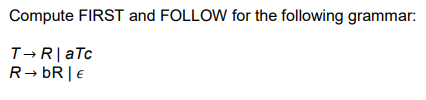Home / Expert Answers / Computer Science / compute-first-and-follow-for-the-following-grammar-begin-array-l-t-ri-pa481

# (Solved): Compute FIRST and FOLLOW for the following grammar: $\begin{array}{l} T \ri ...??????? Compute FIRST and FOLLOW for the following grammar: \[ \begin{array}{l} T \rightarrow R \mid a T c \\ R \rightarrow \mathrm{bR} \mid \epsilon \end{array}$

We have an Answer from Expert